## Model Coverage for MATLAB Functions

### About Model Coverage for MATLAB Functions

The Simulink® Coverage™ software simulates a Simulink model and reports model coverage data for the decisions and conditions of code in MATLAB Function blocks. Model coverage only supports coverage for MATLAB® functions configured for code generation.

For example, consider the following `if` statement:

```if (x > 0 || y > 0) reset = 1; ```

The `if` statement contains a decision with two conditions (`x > 0` and `y > 0`). The Simulink Coverage software verifies that all decisions and conditions are taken during the simulation of the model.

### Types of Model Coverage for MATLAB Functions

The types of model coverage that the Simulink Coverage software records for MATLAB functions configured for code generation are:

#### Decision Coverage

During simulation, the following MATLAB Function block statements are tested for decision coverage:

• Function header — Decision coverage is 100% if the function or local function is executed.

• `if` — Decision coverage is 100% if the `if` expression evaluates to `true` at least once, and `false` at least once.

• `switch` — Decision coverage is 100% if every `switch` case is taken, including the fall-through case.

• `for` — Decision coverage is 100% if the equivalent loop condition evaluates to `true` at least once, and `false` at least once.

• `while` — Decision coverage is 100% if the equivalent loop condition evaluates to `true` at least once, and evaluates to `false` at least once.

#### Condition and MCDC Coverage

During simulation, in the MATLAB Function block function, the following logical conditions are tested for condition and MCDC coverage:

• `if` statement conditions

• Logical expressions in assignment statements

The following MATLAB functions are active in code generation and in Simulink Design Verifier™:

When you specify the Objectives and Constraints coverage metric in the Coverage pane of the Configuration Parameters dialog box, the Simulink Coverage software records coverage for these functions.

Each of these functions evaluates an expression `expr`, for example, `sldv.test(expr)`, where `expr` is a valid Boolean MATLAB expression. Simulink Design Verifier coverage measures the number of time steps that the expression `expr` evaluates to `true`.

If `expr` is `true` for at least one time step, Simulink Design Verifier coverage for that function is 100%. Otherwise, the Simulink Coverage software reports coverage for that function as 0%.

For an example of coverage data for Simulink Design Verifier functions in a coverage report, see Simulink Design Verifier Coverage.

#### Relational Boundary Coverage

If the MATLAB function block contains a relational operation, the relational boundary coverage metric applies to this block.

If the MATLAB function block calls functions containing relational operations multiple times, the relational boundary coverage reports a cumulative result over all instances where the function is called. If a relational operation in the function uses operands of different types in the different calls, relational boundary coverage uses tolerance rules for the stricter operand type. For instance, if a relational operation uses `int32` operands in one call, and `double` operands in another call, relational boundary coverage uses tolerance rules for `double` operands.

For information on the tolerance rules and the order of strictness of types, see Relational Boundary Coverage.

### How to Collect Coverage for MATLAB Functions

When you simulate your model, the Simulink Coverage software can collect coverage data for MATLAB functions configured for code generation. You enable model coverage from the Coverage app.

You collect model coverage for MATLAB functions as follows:

• Functions in a MATLAB Function block

• Functions in an external MATLAB file

To collect coverage for an external MATLAB file, Coverage pane of the Configuration Parameters dialog box, select Coverage for MATLAB files.

To collect coverage for these functions, on the Coverage pane of the Configuration Parameters dialog box, select the Objectives and Constraints coverage metric.

The following section provides model coverage examples for each of these situations.

### Examples: Model Coverage for MATLAB Functions

#### Model Coverage for MATLAB Function Blocks

Simulink Coverage software measures model coverage for functions in a MATLAB Function block.

The following model contains two MATLAB functions in its MATLAB Function block:In the Configuration Parameters dialog box, on the Solver pane, under Solver selection, the simulation parameters are set as follows:

• Type`Fixed-step`

• Solver```discrete (no continuous states)```

• Fixed-step size (fundamental sample time)`1`

The MATLAB Function block contains two functions:

• The top-level function, `run_intersect_test`, sends the coordinates for two rectangles, one fixed and the other moving, as arguments to `rect_intersect`.

• The local function, `rect_intersect`, tests for intersection between the two rectangles. The origin of the moving rectangle increases by `1` in the `x` and `y` directions with each time step.

The coordinates for the origin of the moving test rectangle are represented by persistent data `x1` and `y1`, which are both initialized to `-1`. For the first sample, `x1` and `y1` both increase to `0`. From then on, the progression of rectangle arguments during simulation is as shown in the following graphic.The fixed rectangle is shown in bold with a lower-left origin of `(2,4)` and a width and height of 2. At time ```t = 0```, the first test rectangle has an origin of `(0,0)` and a width and height of 2. For each succeeding sample, the origin of the test rectangle increments by `(1,1)`. The rectangles at sample times `t = 2`, `3`, and `4` intersect with the test rectangle.

The local function `rect_intersect` checks to see if its two rectangle arguments intersect. Each argument consists of coordinates for the lower-left corner of the rectangle (origin), and its width and height. `x` values for the left and right sides and `y` values for the top and bottom are calculated for each rectangle and compared in nested `if-else` decisions. The function returns a logical value of 1 if the rectangles intersect and 0 if they do not.

Scope output during simulation, which plots the return value against the sample time, confirms the intersecting rectangles for sample times `2`, `3`, and `4` .After the simulation, the model coverage report appears in a browser window. After the summary in the report, the Details section of the model coverage report reports on each parts of the model.

The model coverage report for the MATLAB Function block shows that the block itself has no decisions of its own apart from its function.

The following sections examine the model coverage report for the example model in reverse function-block-model order. Reversing the order helps you make sense of the summary information at the top of each section.

Coverage for the MATLAB Function run_intersect_test.  Model coverage for the MATLAB Function block function `run_intersect_test` appears under the linked name of the function. Clicking this link opens the function in the editor.

Below the linked function name is a link to the model coverage report for the parent MATLAB Function block that contains the code for `run_intersect_test`.The top half of the report for the function summarizes its model coverage results. The coverage metrics for `run_intersect_test` include decision, condition, and MCDC coverage. You can best understand these metrics by examining the code for `run_intersect_test`.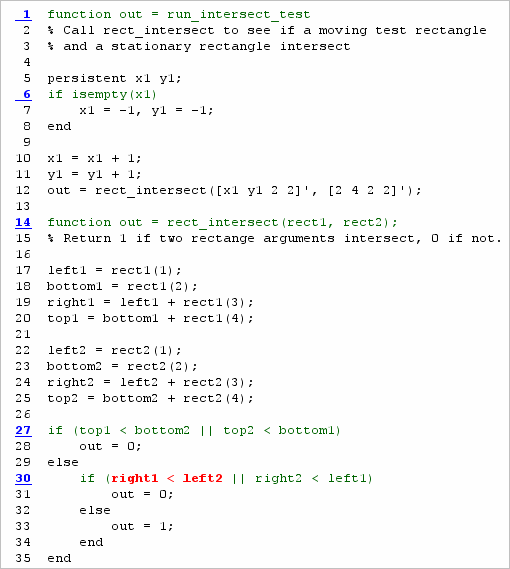Lines with coverage elements are marked by a highlighted line number in the listing:

• Line 1 receives decision coverage on whether the top-level function `run_intersect_test` is executed.

• Line 6 receives decision coverage for its `if` statement.

• Line 14 receives decision coverage on whether the local function `rect_intersect` is executed.

• Lines 27 and 30 receive decision, condition, and MCDC coverage for their `if` statements and conditions.

Each of these lines is the subject of a report that follows the listing.

The condition `right1 < left2` in line 30 is highlighted in red. This means that this condition was not tested for all of its possible outcomes during simulation. Exactly which of the outcomes was not tested is in the report for the decision in line 30.

The following sections display the coverage for each `run_intersect_test` decision line. The coverage for each line is titled with the line itself, which if clicked, opens the editor to the designated line.

Coverage for Line 1.  The coverage metrics for line 1 are part of the coverage data for the function `run_intersect_test`.

The first line of every MATLAB function configured for code generation receives coverage analysis indicative of the decision to run the function in response to a call. Coverage for `run_intersect_test` indicates that it executed at least once during simulation.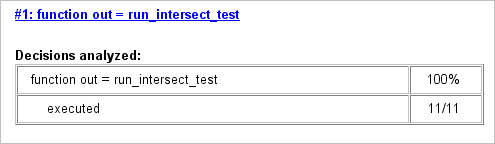Coverage for Line 6.  The Decisions analyzed table indicates that the decision in line 6, `if isempty(x1)`, executed a total of eight times. The first time it executed, the decision evaluated to `true`, enabling `run_intersect_test` to initialize the values of its persistent data. The remaining seven times the decision executed, it evaluated to `false`. Because both possible outcomes occurred, decision coverage is 100%.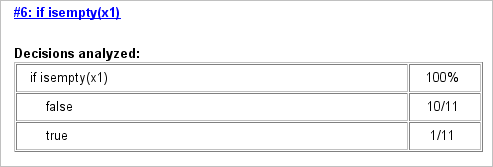Coverage for Line 14.  The Decisions analyzed table indicates that the local function `rect_intersect` executed during testing, thus receiving 100% coverage.Coverage for Line 27.  The Decisions analyzed table indicates that there are two possible outcomes for the decision in line 27: `true` and `false`. Five of the eight times it was executed, the decision evaluated to `false`. The remaining three times, it evaluated to `true`. Because both possible outcomes occurred, decision coverage is 100%.

The Conditions analyzed table sheds some additional light on the decision in line 27. Because this decision consists of two conditions linked by a logical OR (`||`) operation, only one condition must evaluate `true` for the decision to be `true`. If the first condition evaluates to `true`, there is no need to evaluate the second condition. The first condition, ```top1 < bottom2```, was evaluated eight times, and was `true` twice. This means that the second condition was evaluated only six times. In only one case was it `true`, which brings the total `true` occurrences for the decision to three, as reported in the Decisions analyzed table.

MCDC coverage looks for decision reversals that occur because one condition outcome changes from `T` to `F` or from `F` to `T`. The MCDC analysis table identifies all possible combinations of outcomes for the conditions that lead to a reversal in the decision. The character `x` is used to indicate a condition outcome that is irrelevant to the decision reversal. Decision-reversing condition outcomes that are not achieved during simulation are marked with a set of parentheses. There are no parentheses, therefore all decision-reversing outcomes occurred and MCDC coverage is complete for the decision in line 27.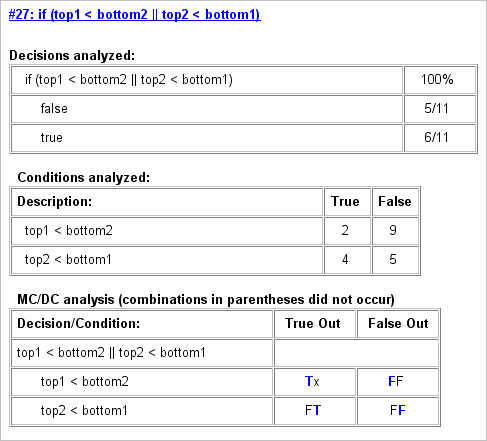Coverage for Line 30.  The line 30 decision, ```if (right1 < left2 || right2 < left1)```, is nested in the `if` statement of the line 27 decision and is evaluated only if the line 27 decision is `false`. Because the line 27 decision evaluated `false` five times, line 30 is evaluated five times, three of which are `false`. Because both the `true` and `false` outcomes are achieved, decision coverage for line 30 is 100%.

Because line 30, like line 27, has two conditions related by a logical OR operator (`||`), condition 2 is tested only if condition 1 is `false`. Because condition 1 tests `false` five times, condition 2 is tested five times. Of these, condition 2 tests `true` two times and `false` three times, which accounts for the two occurrences of the `true` outcome for this decision.

Because the first condition of the line 30 decision does not test `true`, both outcomes do not occur for that condition and the condition coverage for the first condition is highlighted with a rose color. MCDC coverage is also highlighted in the same way for a decision reversal based on the `true` outcome for that condition.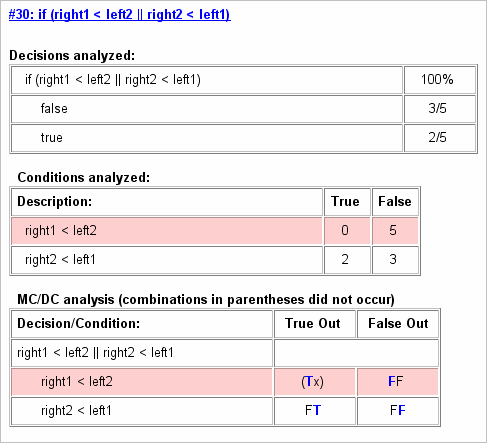Coverage for run_intersect_test.  On the Details tab, the metrics that summarize coverage for the entire `run_intersect_test` function are reported and repeated as shown.The results summarized in the coverage metrics summary can be expressed in the following conclusions:

• There are eight decision outcomes reported for `run_intersect_test` in the line reports:

• One for line 1 (executed)

• Two for line 6 (`true` and `false`)

• One for line 14 (executed)

• Two for line 27 (`true` and `false`)

• Two for line 30 (`true` and `false`).

The decision coverage for each line shows 100% decision coverage. This means that decision coverage for `run_intersect_test` is eight of eight possible outcomes, or 100%.

• There are four conditions reported for `run_intersect_test` in the line reports. Lines 27 and 30 each have two conditions, and each condition has two condition outcomes (`true` and `false`), for a total of eight condition outcomes in `run_intersect_test`. All conditions tested positive for both the `true` and `false` outcomes except the first condition of line 30 (```right1 < left2```). This means that condition coverage for `run_intersect_test` is seven of eight, or 88%.

• The MCDC coverage tables for decision lines 27 and 30 each list two cases of decision reversal for each condition, for a total of four possible reversals. Only the decision reversal for a change in the evaluation of the condition `right1 < left2` of line 30 from `true` to `false` did not occur during simulation. This means that three of four, or 75% of the possible reversal cases were tested for during simulation, for a coverage of 75%.

#### Model Coverage for MATLAB Functions in an External File

Using the same model in Model Coverage for MATLAB Function Blocks, suppose the MATLAB functions `run_intersect_test` and `rect_intersect` are stored in an external MATLAB file named `run_intersect_test.m`.

To collect coverage for MATLAB functions in an external file, on the Coverage pane of the Configuration Parameters dialog box, select Coverage for MATLAB files.

After simulation, the model coverage report summary contains sections for the top-level model and for the external function.The model coverage report for `run_intersect_test.m` reports the same coverage data as if the functions were stored in the MATLAB Function block.

For a detailed example of a model coverage report for a MATLAB function in an external file, see External MATLAB File Coverage Report.

#### Model Coverage for Simulink Design VerifierMATLAB Functions

If the MATLAB code includes any of the following Simulink Design Verifier functions configured for code generation, you can measure coverage:

For this example, consider the following model that contains a MATLAB Function block.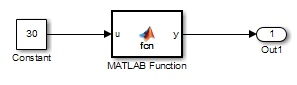The MATLAB Function block contains the following code:

```function y = fcn(u) % This block supports MATLAB for code generation. sldv.condition(u > -30) sldv.test(u == 30) y = 1;```

To collect coverage for Simulink Design Verifier MATLAB functions, on the Coverage pane in the Configuration Parameters dialog box, under Other metrics, select Objectives and Constraints.

After simulation, the model coverage report listed coverage for the `sldv.condition` and `sldv.test` functions. For `sldv.condition`, the expression ```u > -30``` evaluated to `true` 51 times. For `sldv.test`, the expression `u == 30` evaluated to `true` 51 times.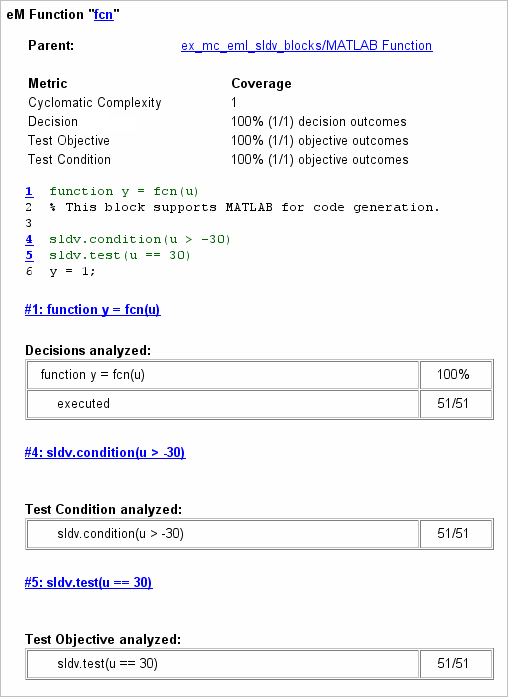For an example of model coverage data for Simulink Design Verifier blocks, see Objectives and Constraints Coverage.